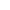What are the limitations of Ohm's Law? Physics Q&A
Question

# What are the limitations of Ohm's Law?

Open in AppSolution

## Ohm's law:Ohm’s law states that the electric current is proportional to the voltage and is inversely proportional to the resistance.Mathematically, this current-voltage relationship is written as,$\mathrm{V}=\mathrm{IR}$Here, V is voltage.I is current.R is resistance.The same formula can be written in the order to calculate resistance and current as follows:$\mathrm{I}=\frac{\mathrm{V}}{\mathrm{R}}$$\mathrm{R}=\frac{\mathrm{V}}{\mathrm{I}}$Limitations of ohm's law:Ohm's law does not apply to unilateral electrical components such as diodes as well as transistors even though they only permit current just to flow in one way.Voltage level will not be constant with respect to time for non-linear electrical components with properties such as capacitance, resistance, and so on, making Ohm's law problematic to apply.Example:A water volt-ammeter. It is an example of a unilateral network and Ohm’s law is not applicable to such networks.Semiconductors such as Silicon and Germanium don’t obey Ohm’s law and are known as Non-Ohmic conductors.Suggest Corrections1Similar questions